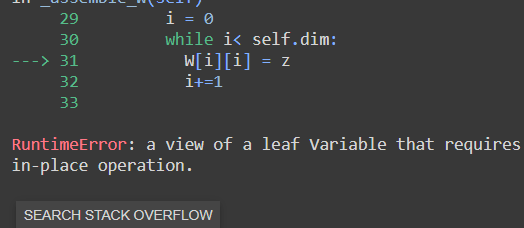# How to create a custom parameter?

I want to create a parameter v which is a vector and make sure that it is initialized to values that are non-zero. How do I do that?

You could create a tensor with values drawn from a Gaussian distribution and wrap it in an `nn.Parameter` as seen here:

``````param = nn.Parameter(torch.randn(size))
``````
1 Like

So when the parameter updates will it only take values from randn? Also since 0 is one of the values in randn , how to make sure that none of the values in the tensor is 0?

No, since the gradient determines the updates, not the initial values.

The probability to sample a “perfect” `0.0` value is not very high, but in case you want to make sure no zeros are there, you could replace them before creating the parameter:

``````x = torch.randn(10)
x = 0.0
zero_idx = x==0.0
while zero_idx.any():
x[zero_idx] = torch.randn_like(x[zero_idx])
zero_idx = x==0.0

param = nn.Parameter(x)
``````
1 Like

thanks. please could I also know how to make sure that the parameter values lie between -2000 and 1500 during gradient updates?

You could `.clamp_` the parameter after each gradient update to make sure it’s in the desired value range.

1 Like

I have a custom parameter `W = nn.Parameter(torch.randn(1))` When i try to do `W += 2` or when i try to do `W.data += 2` , I get the following error:
`RuntimeError: a leaf Variable that requires grad is being used in an in-place operation.`
Please help
please tell me how to do inplace operations on custom parameters

If the parameter value is needed for the gradient computation, an inplace operation is disallowed, as explained in the error message.
In such a case, remove the inplace operation and use the out-of-place one:

``````x = x + 2
``````

Also, don’t use the `.data` attribute, as it might yield unwanted side effects.

1 Like

Its not working for a 2d parameter W`RuntimeError: a view of a leaf Variable that requires grad is being used in an in-place operation.`
Here,
`z = torch.zeros(1)`
Please help